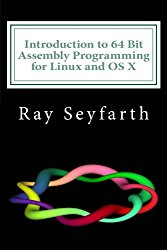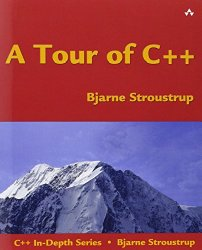Writing a minimal x86-64 JIT compiler in C++ - Part 2

Posted on January 12, 2018 by Paul

In my last article, I’ve shown you how to generate the machine code for a function at runtime, copy this code in a part of the memory, marked as executable, and call it from C++. Now, we’ll go the other way around, we’ll call a C++ function from a function generated at runtime. Like before, I assume that you try the code on Linux or macOS.

If you remember from part 1, we’ve started by adding machine code instructions in an std::vector and copying this code to an executable memory page. While this was a fine approach from a didactic point of view, in practice, you will probably want to write the code directly to the executable memory. Here is an example of how I propose to do it:

The object mp, from the above piece of code, will ask the OS for memory, release this memory when it is not needed and will have some helper member functions that will let us push pieces of machine code to the executable memory. We can also add safety features, e.g. a mechanism to check if we can push more data on the executable memory or if we’ve reached the bounds of the allocated memory pages.

For simplicity, I will keep the entire code of this example in a single source file. We can split it later in more files if it grows too big.

Let’s start by writing the code for MemoryPages:

In the above, position points to the beginning of the non used memory area. This grows as we push more machine code to the executable memory.

Next, we basically copy the code that asks for executable memory from the previous tutorial to the struct constructor and the code that releases this memory to the destructor:

Please note that by default the constructor will allocate a single page of memory, pass the required number of pages if you need more. If you want to use this example in production, I suggest to implement a mechanism to ask for more memory pages only when needed, see the mremap documentation for hints. For our purposes, one page of memory is more than enough and will keep the code simpler.

Implementing a member function that pushes a byte of data to the memory is straightforward:

Last time, we’ve used an explicit approach to push numbers larger than one byte to the memory for didactic purposes (by manually extracting the bytes from the input number and adding them one by one in reverse order, as is the default for little-endian byte order of the Intel processor). It is more efficient and less error prone to use a function like std::memcpy to copy the individual bytes of a larger number, in the correct byte order, for the machine on which the code runs. We’ll use memcpy to copy the address of a function pointer to the memory in the next push function:

In some cases, the machine code for a particular set of instructions is just a set of bytes, e.g.:

is translated to machine code as:

it could be useful to have another push function that will receive as input a std::vector of uint8_t numbers:

The code that checks if we can copy some data to the memory:

As suggested earlier, if you intend to use this code in production, it will be a good idea to use mremap to ask for more memory pages, if necessary, and throw an error only if the OS can’t satisfy the demand.

Finally, we could add a helper function to print the content of the occupied memory:

Now, that we’ve finished abstracting the main ideas from the previous article, we can get to the juicy bits of the current one - calling an existing C++ function from our generated machine code at runtime. Let’s simplify a bit the problem and investigate how we can call a C++ function that receives no argument and returns nothing.

OK, so let’s write a C++ function that prints a message and (bare with me) modifies a global variable. I know that using globals is usually a bad practice, but it will allow me to illustrate that calling a C++ function from our code generated at runtime can have side effects. It will also be useful for the next article in this series, that will implement a mini Forth interpreter that can JIT compile user defined functions.

The idea is to call the function test() from a function generated at runtime, say func. This is how our func function could look in Assembly:

We can further simplify the above code by converting line 5 from above to:

The body of the above function can look like this in machine code:

In the above code, the first two lines of the function body are called prologue and the last two lines are called epilogue and they are, by convention, repeated in all new functions. You can read more here. It make sense to put these two chunks of machine code in two separate variables and use these when we need them:

Next, we can write the main program. First, we create an instance of MemoryPages and we push the required machine code:

If we run the above code, we should see the generated machine code. Here is what I see on a macOS machine:

At this point, all we have to do is to cast the address of our generated code to a function pointer and call the function. We’ll also show the side effects of calling test() on the global variable a:

This is what I see on a macOS machine:

If you run the code on your machine, you should get identical results, except for the machine code part that stores the address of the C++ function.

You can find the complete source code for the above example on the GitHub repo for this article.

If you are interested to learn more about x86-64 Assembly, I would recommend reading Introduction to 64 bit Assembly Programming for Linux and OS X by Ray Seyfarth:If you are interested to learn more about modern C++, A Tour of C++ by Bjarne Stroustrup is a decent introduction: